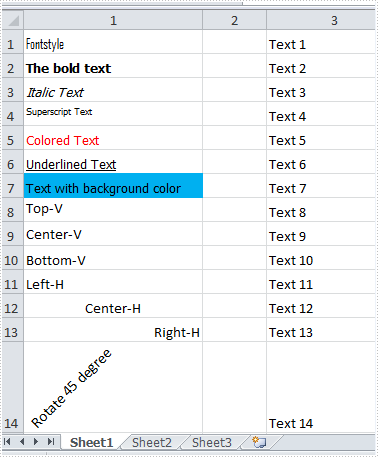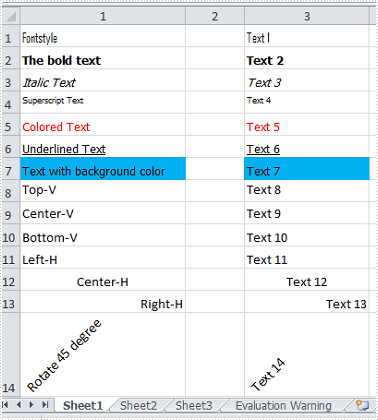# Copy Formatting from One Cell or Range to Another in C#, VB.NET

When we are dealing with Excel spreadsheets, we may get a cell or a range of cells formatted, then we can use the Format Painter to quickly copy formatting from one place and apply it to another. It saves us much time to do so if we want to format a cell or cells with the previous cell formatting. In this article, I'll introduce you how to copy formatting from a range of cells to another using Spire.XLS.

As is shown in the screenshot of test file, contents in the first column have been formatted with different styles, what we want is to correspondingly apply cell formatting from column 1 to column 3.#### Code snippets:

Step 1: Create a new instance of Wordbook class and load the test file from disk.

```       Workbook workbook = new Workbook();
```

Step 2: Get the worksheet from workbook.

```       Worksheet sheet = workbook.Worksheets;
```

Step 3: Copy the cell formatting from column 1 and apply to cells of column 3.

```       int count = sheet.Rows.Count();
for (int i = 1; i < count + 1; i++)
{
sheet.Range[string.Format("C{0}", i)].Style = sheet.Range[string.Format("A{0}", i)].Style;
}
```

Step 4: Save to a new Excel file.

```        workbook.SaveToFile("Result.xlsx", ExcelVersion.Version2010);
```

Result:Full Code:

[C#]
```           static void Main(string[] args)
{
Workbook workbook = new Workbook();
Worksheet sheet = workbook.Worksheets;
int count = sheet.Rows.Count();
for (int i = 1; i < count + 1; i++)
{
sheet.Range[string.Format("C{0}", i)].Style = sheet.Range[string.Format("A{0}", i)].Style;
}
workbook.SaveToFile("Result.xlsx", ExcelVersion.Version2010);
}
```
[VB.NET]
```       Private Shared Sub Main(args As String())
Dim workbook As New Workbook()